# Andrew Martinho’s Market Overview

## Weekly strategy for March 04 – 09, 2013

CONFUSED about where to enter your trades I have put together a series of pivot reviews using fractal daily markers.  I hope it helps out.  Please get in contact with me about SOCIAL INDEX’s here in etoro and i will explain how you can follow these index’s and diversify even more.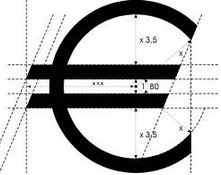EUR/JPY

Last week the EUR JPY pair had a bullish rally on Friday, as 120 yen level offered support to pair. If you look at the weekly chart, this pair is within a short-term bearish channel and it is likely that this week it will make attempts to break this level. But for the long term this pair has behaved extremely bullish. Remember that the Bank of Japan is aimed at weakening the yen. Therefore, for this new week of the month, we have the pivot level, where we recommend buying if the pair closes above 121.95. On the other hand, if the pair trades below the weekly pivot, you can sell this pair. For it to succeed in trading, you should be very attentive to the levels of 1.30 and 1.3250, the price of the EUR/USD pair. We have outlined the likely movements and signals, they can be found below. 121.94 and 122.44 are weekly and monthly pivot points for this week for EUR/JPY.

Signals for March 04 – 09, 2013

Buy above 121.94 with take profit at 125.10 and 128.35 (W_R2), stop loss is below 118.69 (W_S1). Sell if it closes below 121.80 (W_PPV) with take profit at 118.69 (W_S1), stop loss is above 122.50.

____WEEKLY_____

1. Weekly – R3 = 131.51
2. Weekly – R2 = 128.35
3. Weekly – R1 = 125.10
4. Weekly Pivot = 121.94
5. Weekly – S1 = 118.69
6. Weekly – S2 = 115.53
7. Weekly – S3 = 112.28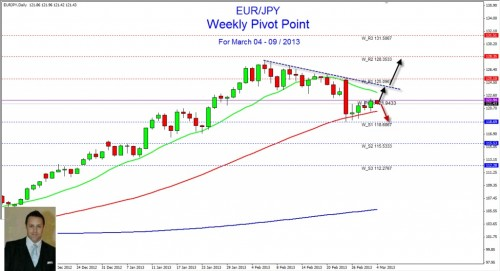MONTHLY

1. Monthly – R3 = 134.99
2. Monthly – R2 = 131.34
3. Monthly – R1 = 126.09
4. Monthly Pivot = 122.44
5. Monthly – S1 = 117.19
6. Monthly – S2 = 113.54
7. Monthly – S3 = 108.29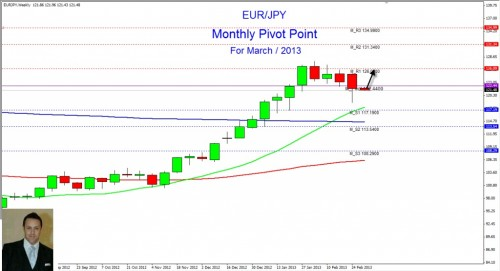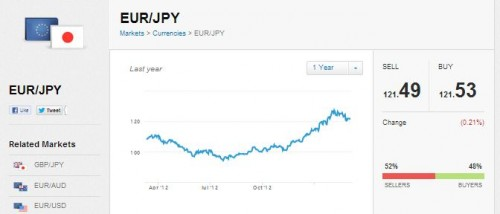Etoro Traders on this instrument are divided down the middle and are unsure of which way the volatile cross is moving.  My gut feeling tells me that BOJ will weaken their currency even more and send the instrument higher.

## AUD/USD

This week the Aussie is near 1.0146 which is support. This level has served as a very strong support in previous months. We believe that in this area the pair will find a break. Tacking it into account, we recommend buying the Aussie in this weekly support level. On the other hand, a breakdown below this level could be quite strong. Therefore, a daily close may be below 1.0140, the fall could be fast until the parity of 1.0000. Therefore, we outline the next signal to be valid for this week.

Signals for March 04 – 09, 2013

Buy above, if it rebounds 1.0146 with take profit at 1.0237 (W_PPV), stop loss is below 1.0120.

_____WEEKLY_____

1. Weekly – R3 = 1.0440
2. Weekly – R2 = 1.0384
3. Weekly – R1 = 1.0293
4. Weekly Pivot = 1.0237
5. Weekly – S1 = 1.0146
6. Weekly – S2 = 1.0090
7. Weekly – S3 = 0.9999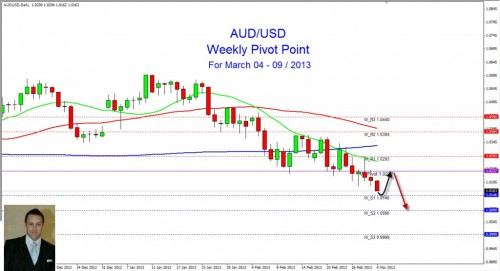MONTHLY____

1. Monthly – R3 = 1.0660
2. Monthly – R2 = 1.0558
3. Monthly – R1 = 1.0386
4. Monthly Pivot = 1.0284
5. Monthly – S1 = 1.0112
6. Monthly – S2 = 1.0010
7. Monthly – S3 = 0.9838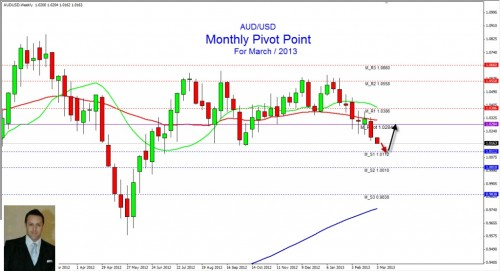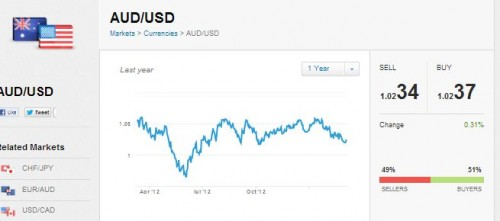As with the JPY Etoro Traders sentiment is divided down the middle, there is equally as much downside potential as there is upside.  Keep an eye on RBA Interest rate decisions and data released it should really stir the market up.  PLEASE COMMENT ON MY WALL AND LET ME KNOW YOUR THOUGHTS.

## GBP/USD

The British pound seems to have been in serious trouble for the last two weeks. The market has had a sharp drop from the level of 1.55 and it seems that this pair is ready to go lower. The psychological level of 1.50 is proving to be a support so far, but if the market drops below weekly support level, 1.4941, the fall would be quick to the level of 1.47. The market is going to have a bounce in this area, if that happens we sell at weekly resistance levels. In the course of the week if the pair closes above the weekly pivot, you can buy with objectives to the resistance level of 1.5315.

Signals for March 04 – 09, 2013

Sell if pullback is around 1.5081 with take profit at 1.4941, stop loss is above 1.51. Buy if close is above 1.5085 with take profit at 1.5175 and 1.5315 (W_R2), stop loss is below 1.4941 (W_S1).

____WEEKLY_____

1. Weekly – R3 = 1.5409
2. Weekly – R2 = 1.5315
3. Weekly – R1 = 1.5175
4. Weekly Pivot = 1.5081
5. Weekly – S1 = 1.4941
6. Weekly – S2 = 1.4847
7. Weekly – S3 = 1.4707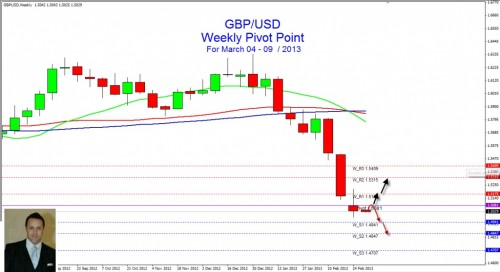_____MONTHLY____

1. Monthly – R3 = 1.6475
2. Monthly – R2 = 1.6176
3. Monthly – R1 = 1.5670
4. Monthly Pivot = 1.5371
5. Monthly – S1 = 1.4865
6. Monthly – S2 = 1.4566
7. Monthly – S3 = 1.4060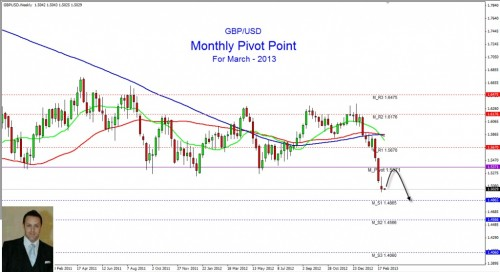## EUR/USD

The euro has managed to close the week above the psychological level of 1.30. If you remember this level has proven to be key in defining the trend of the pair in previous months. Now this pair is trading below the weekly pivot, we expect a strong top on the level of 1.31. If the pair breaks this level, the way is free to the resistance level of 1.3235. If you look at the graph, the euro is in a short-term bearish channel, it is likely to try to break this level in the coming days. On the other hand, if the pair closes below 1.30 level, the pair would fall to 1.2883, first weekly support. We consider that this signal will have validity for the whole week.

Signals for March 04 – 09, 2013

Buy in case of close above 1.3101 (W_PPV), take profit is at 1.3235, and stop loss is below weekly pivot (W_PV) at 1.3050. Sell in case of pullback at 1.31, take profit is at 1.2883, and stop loss is above 1.3150 (W_PV).
____WEEKLY_______

1. Weekly – R3 = 1.3587
2. Weekly – R2 = 1.3553
3. Weekly – R1 = 1.3235
4. Weekly Pivot = 1.3101
5. Weekly – S1 = 1.2883
6. Weekly – S2 = 1.2749
7. Weekly – S3 = 1.2531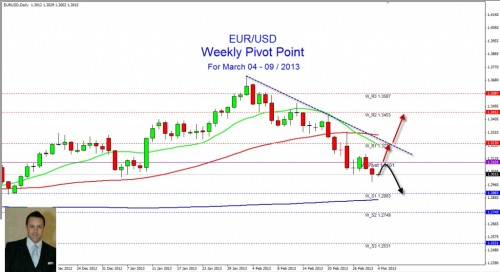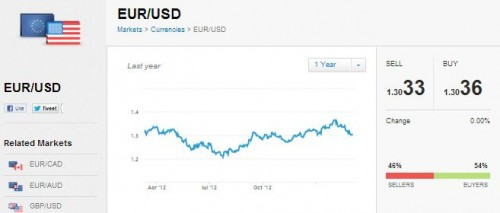Yanalz point of view on the EURO – please comment on my wall and his if you agree or disagree.

MONTHLY____

1. Monthly – R3 = 1.4198
2. Monthly – R2 = 1.3954
3. Monthly – R1 = 1.3505
4. Monthly Pivot = 1.3261
5. Monthly – S1 = 1.2812
6. Monthly – S2 = 1.2568
7. Monthly – S3 = 1.2119

I WISH ALL OF YOU SUCCESSFUL TRADING!!!!!!!!!!!!

Andrew E Martinho | Asian Pacific Rim Region Manager | eToro.com|

Openbook: andrewmaetoro

Email:  andrewma@etoro.com

Skype:andrew.martinho